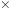### Create an Account

Home / Questions / The total rate at which power used by humans worldwide is approximately 15 TW (terawatts)....

# The total rate at which power used by humans worldwide is approximately 15 TW (terawatts). The solar flux averaged over the sunlit half of Earth is

The total rate at which power used by humans worldwide is approximately 15 TW (terawatts). The solar flux averaged over the sunlit half of Earth is 680 W/m2. (assuming no clouds). The area of Earth’s disc as seen from the sun is 1.281014 m2. The surface area of Earth is approximately 197,000,000 square miles. How much of Earth’s surface would we need to cover with solar energy collectors to power the planet for use by all humans? Assume that the solar energy collectors can convert only 10% of the available sunlight into useful power.

Aug 23 2021 View more View LessSubscribe To Get Solution